# MSBSHSE Solutions For SSC (Class 10) Maths Part 2 Chapter 5 - Coordinate Geometry

MSBSHSE Solutions For SSC (Class 10) Maths Part 2 Chapter 5 – Coordinate Geometry are given here with step by step explanations of each and every solution. BYJU’S provides you error free solutions, which are prepared by our specialized professionals. These solutions help students not only to strengthen their foundation in the subject but also to crack different types of problems easily. This chapter deals with finding the distance between two points and property of intercepts made by three parallel lines. We also come across section formula and midpoint formula in this chapter. Students can easily access these Maharashtra State Board Class 10 Solutions at BYJU’S and download them in PDF format for free.

## Download the PDF of Maharashtra Solutions For SSC Maths Part 2 Chapter 5 Coordinate Geometry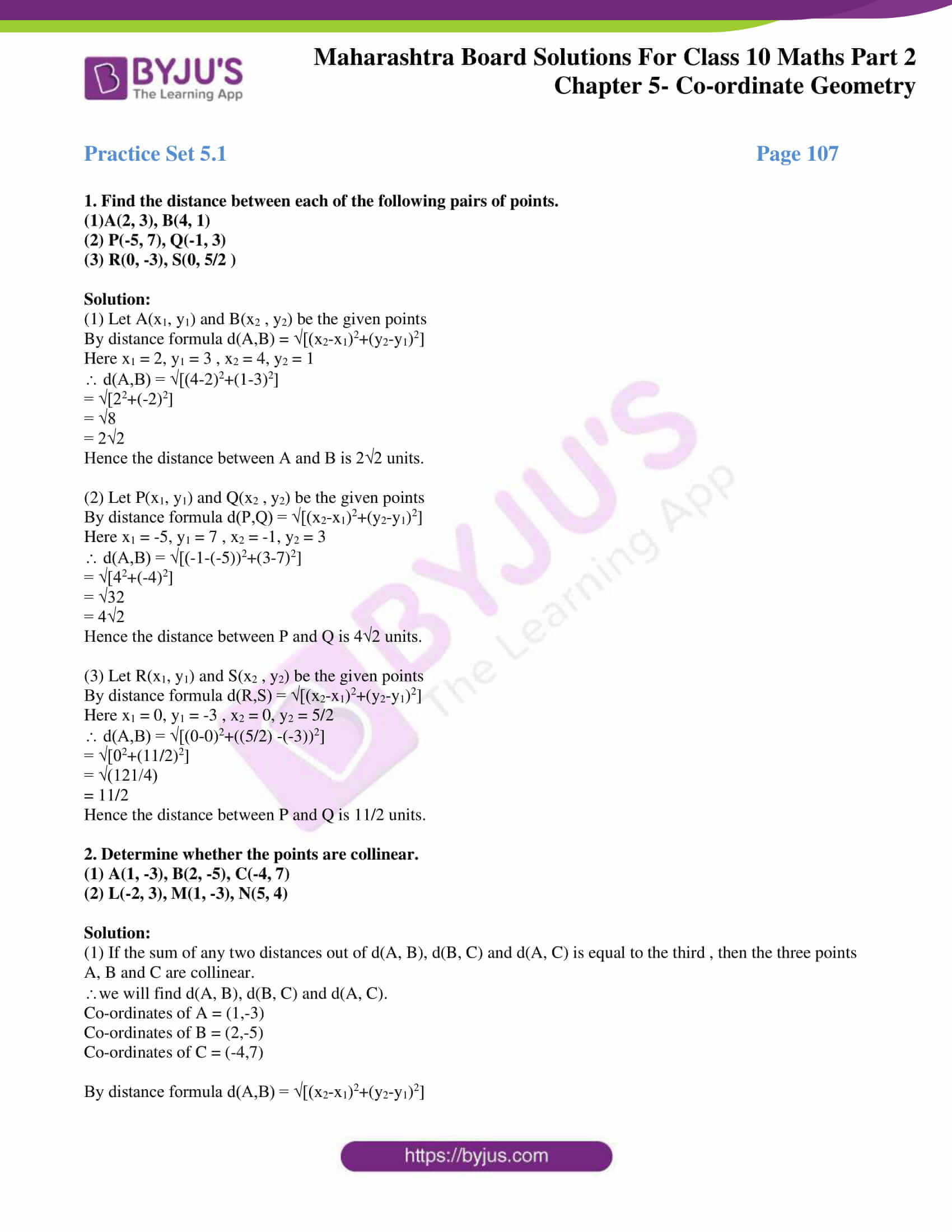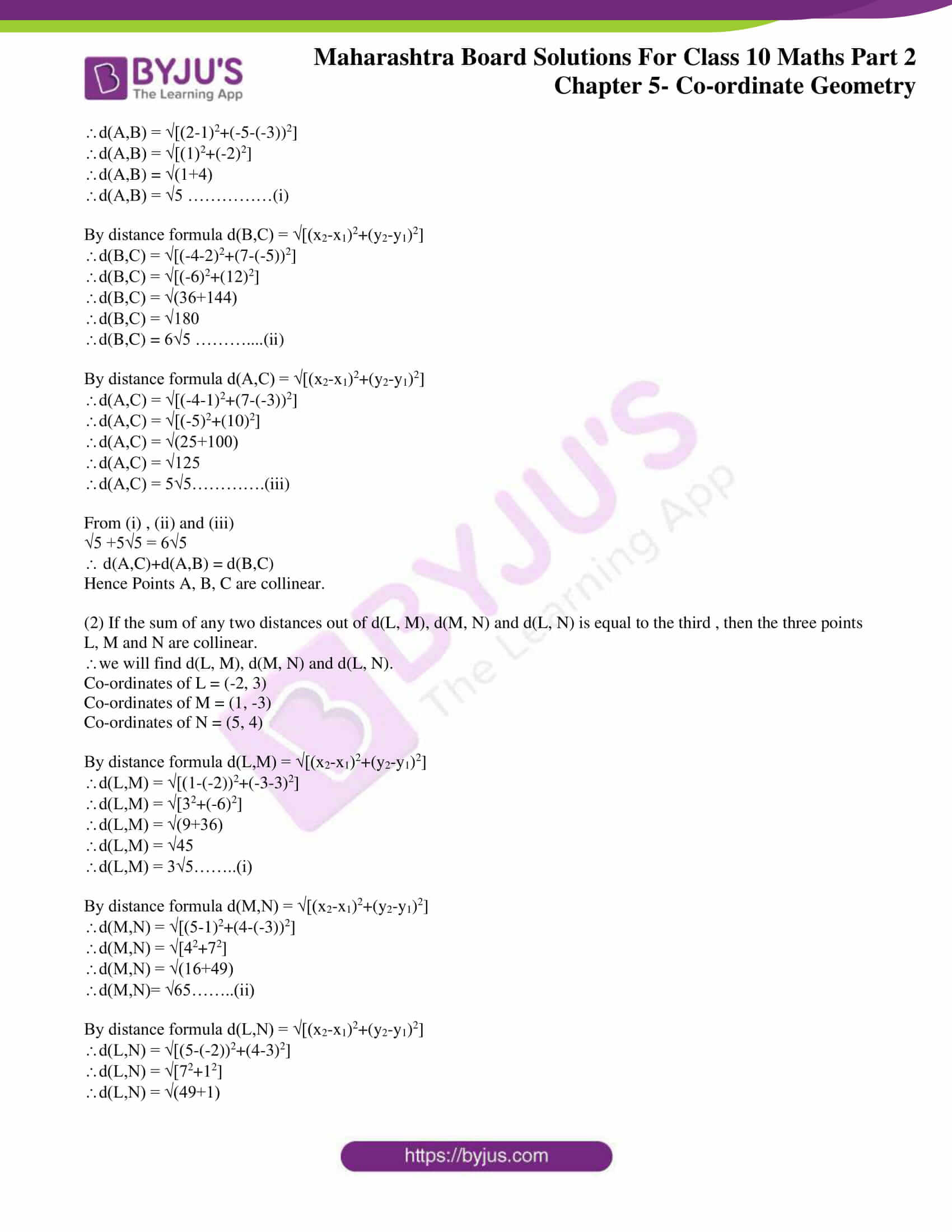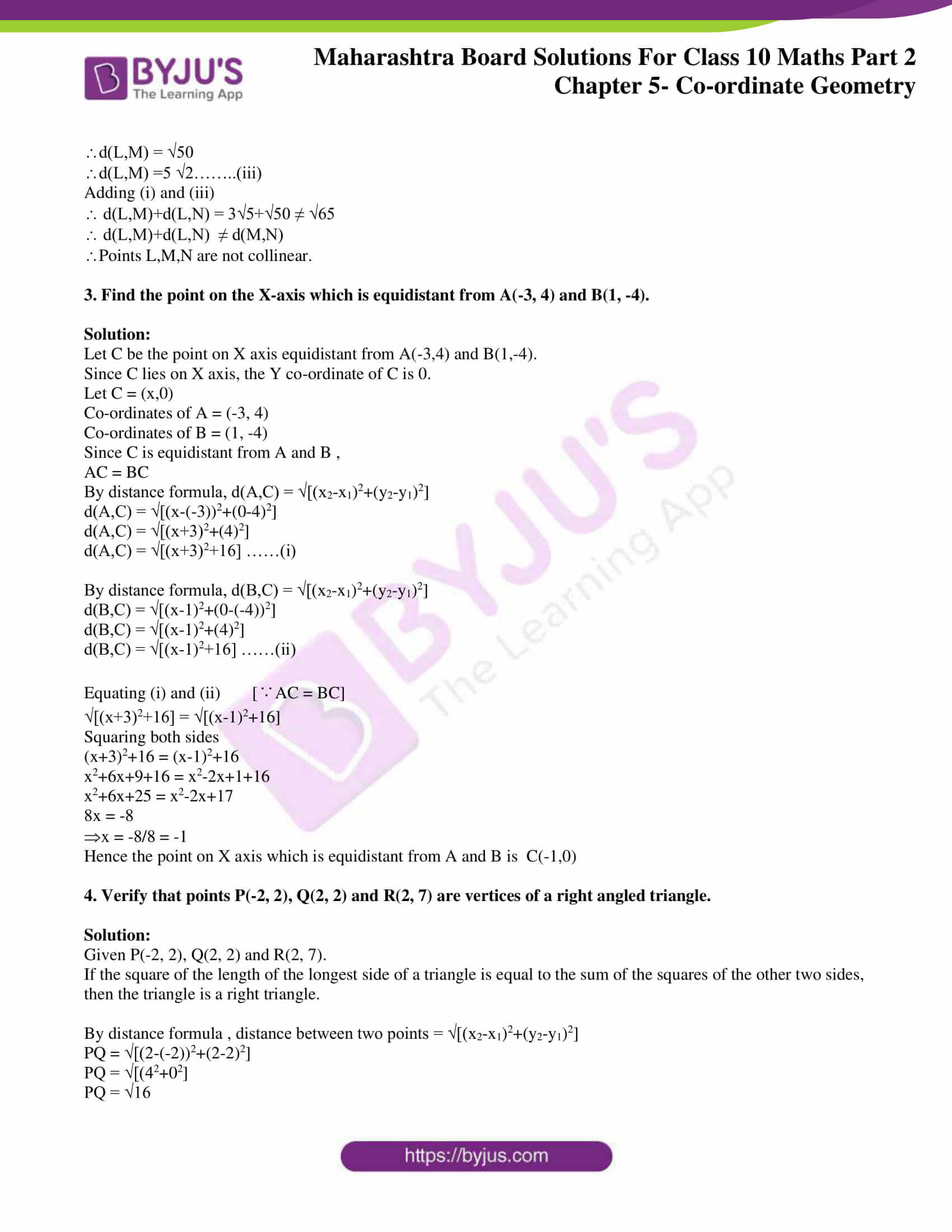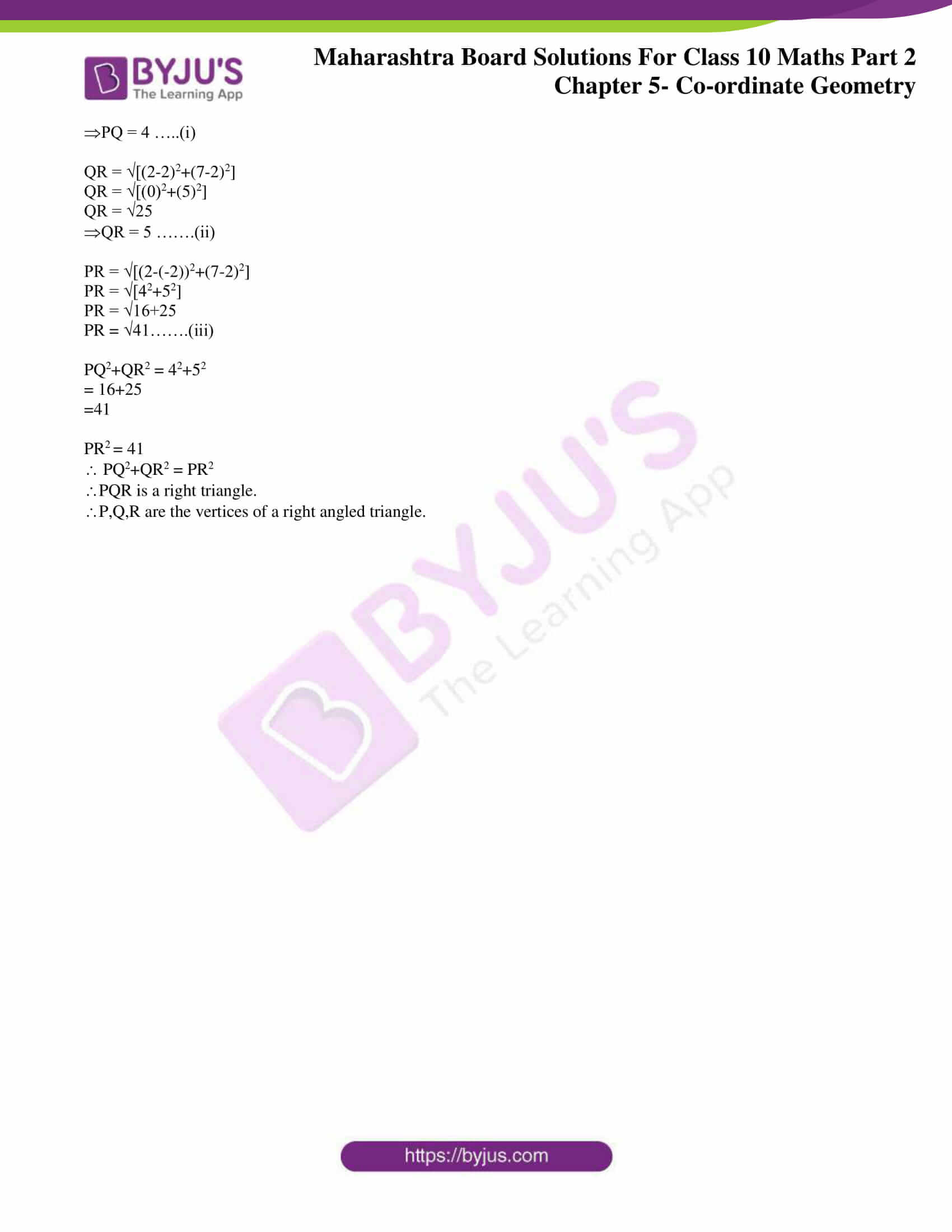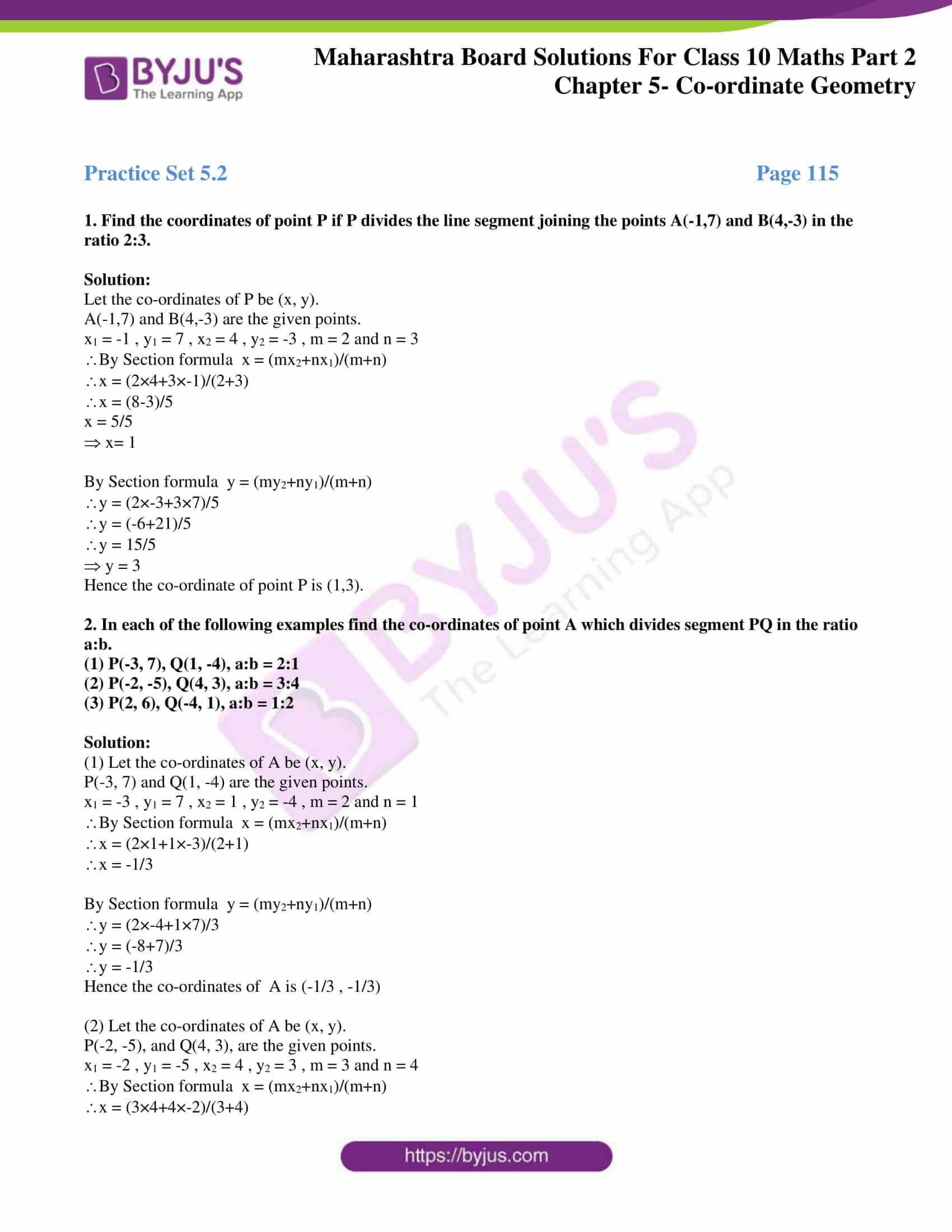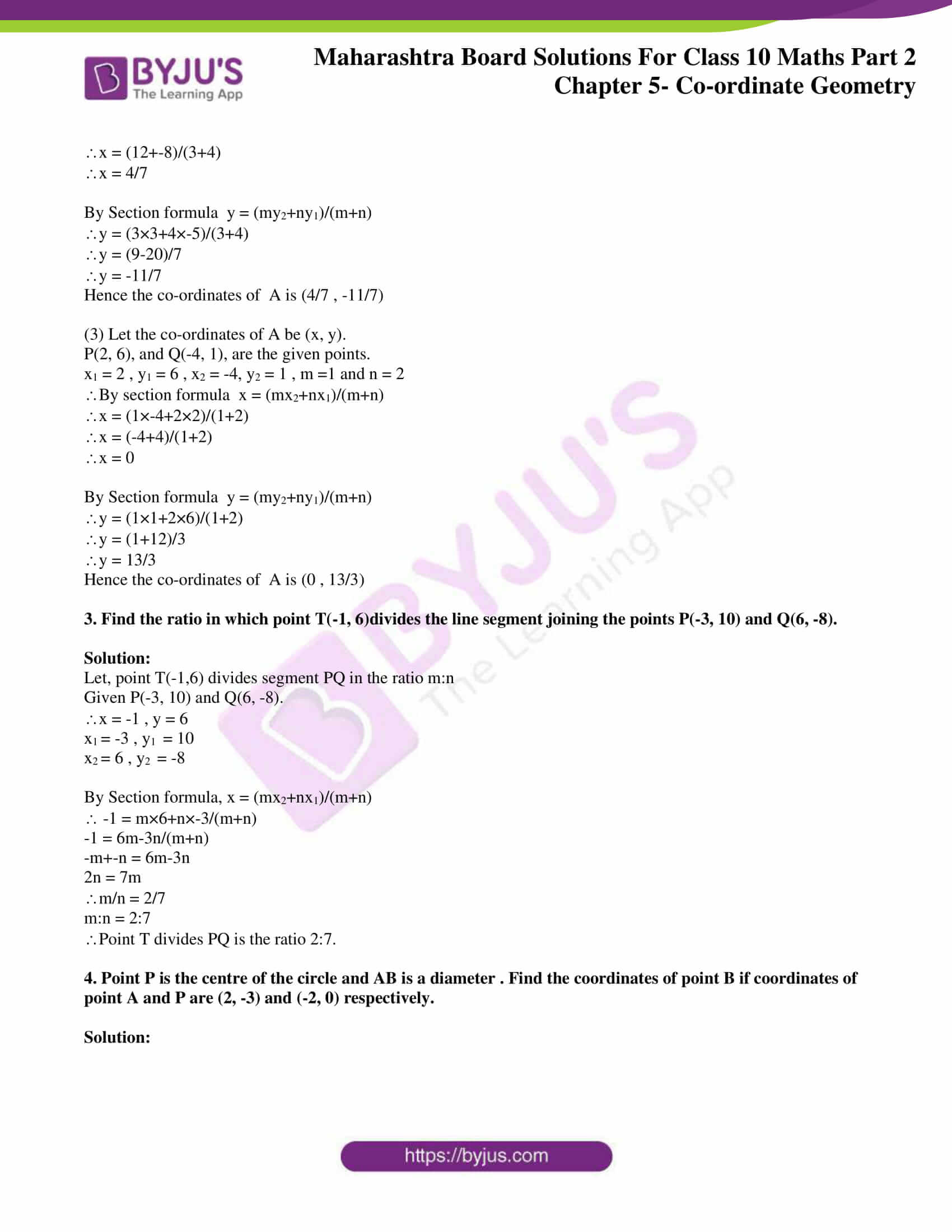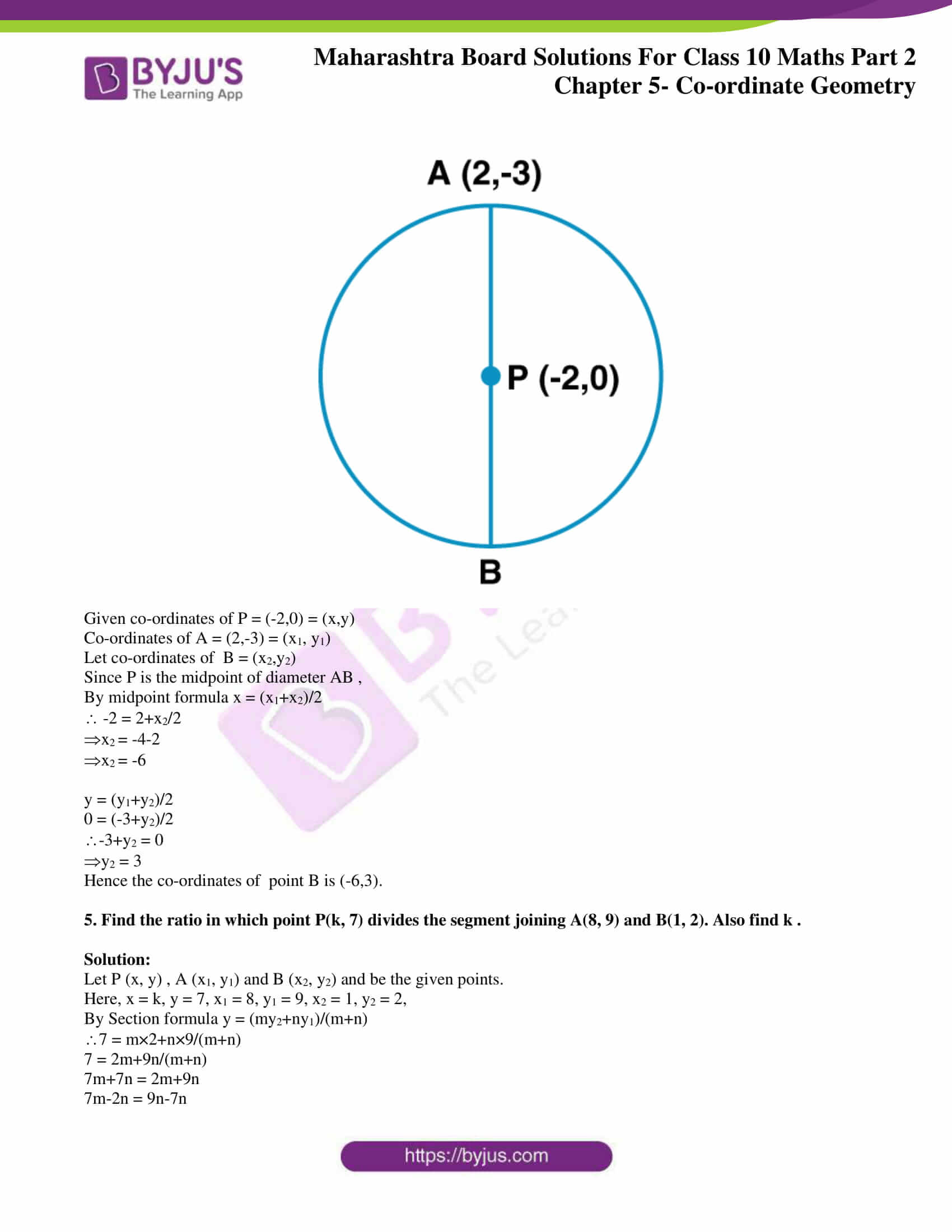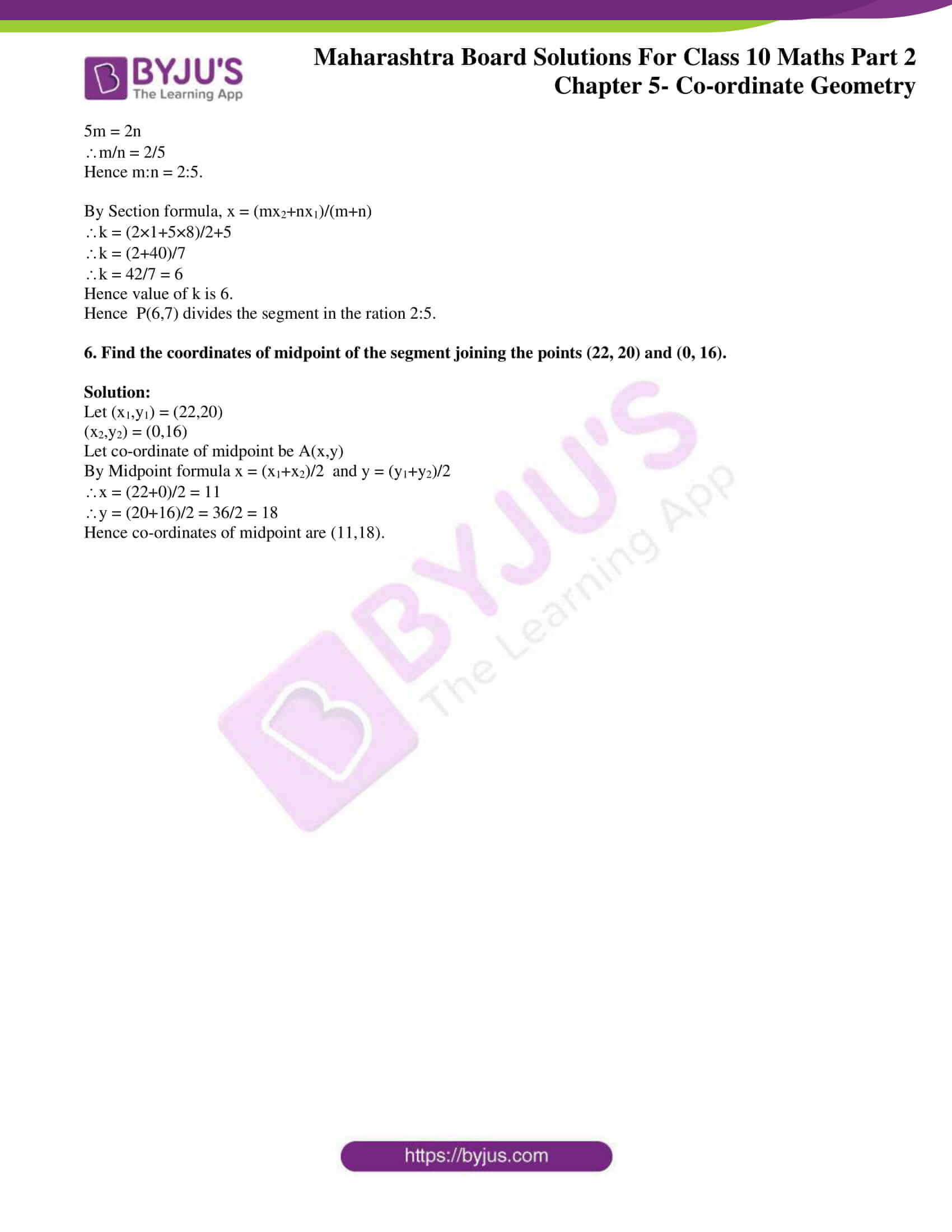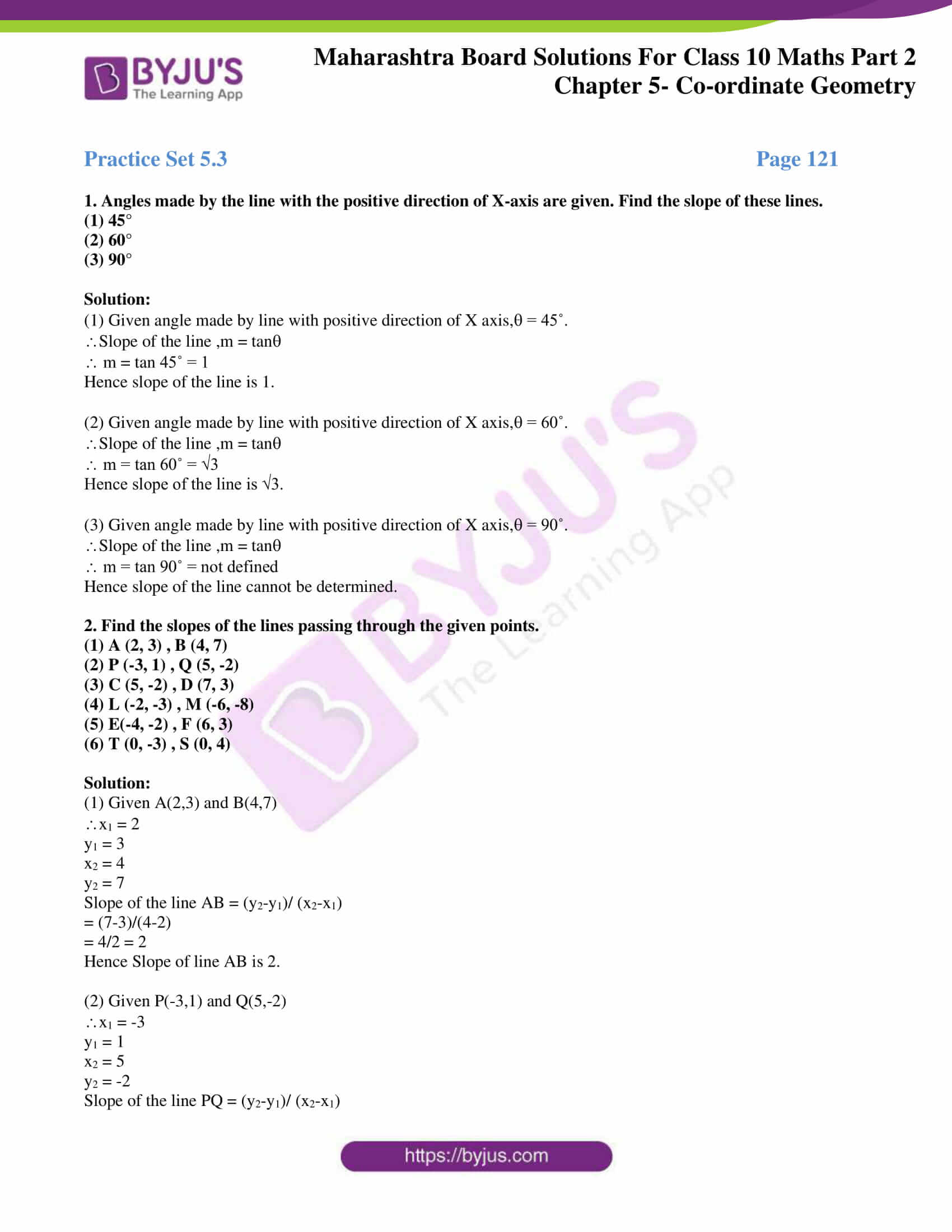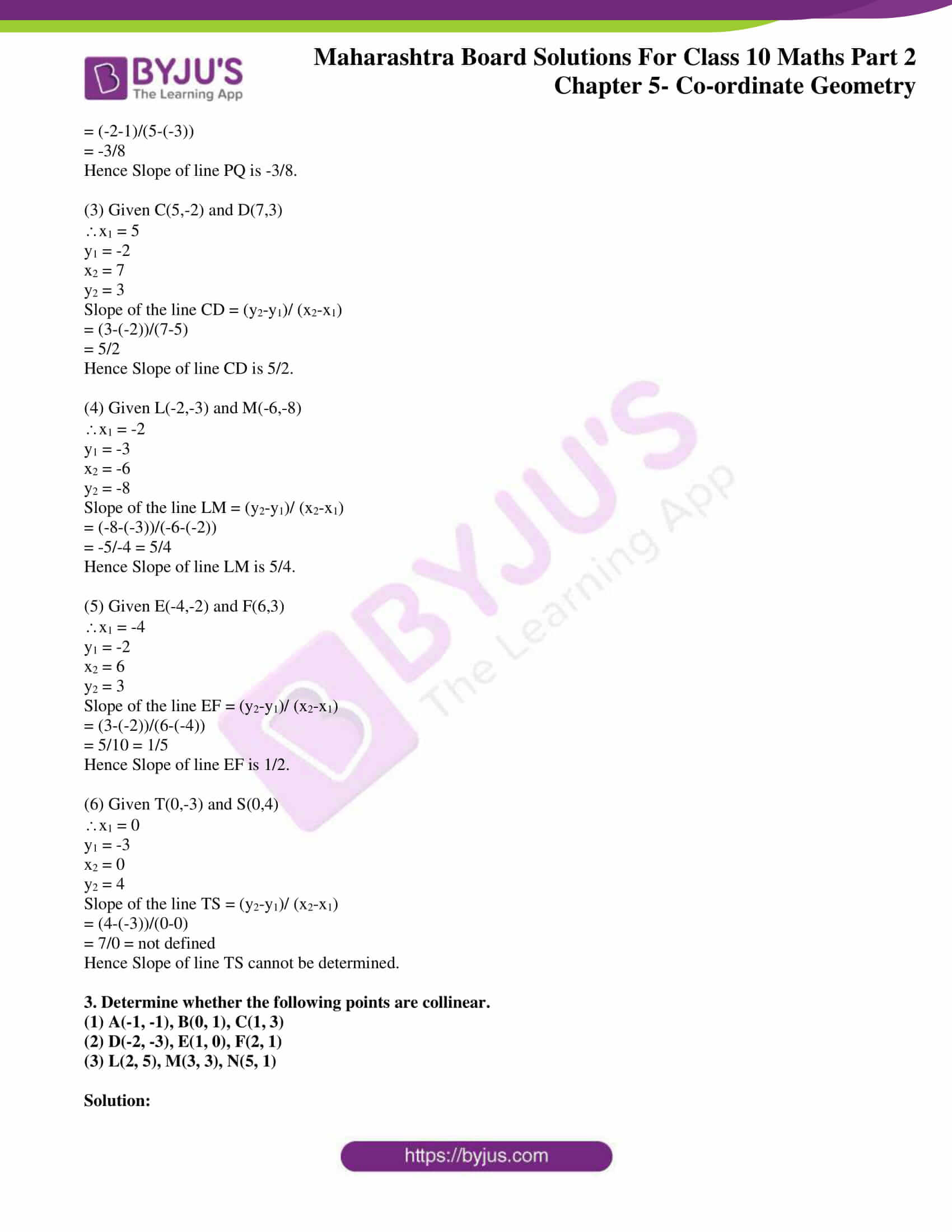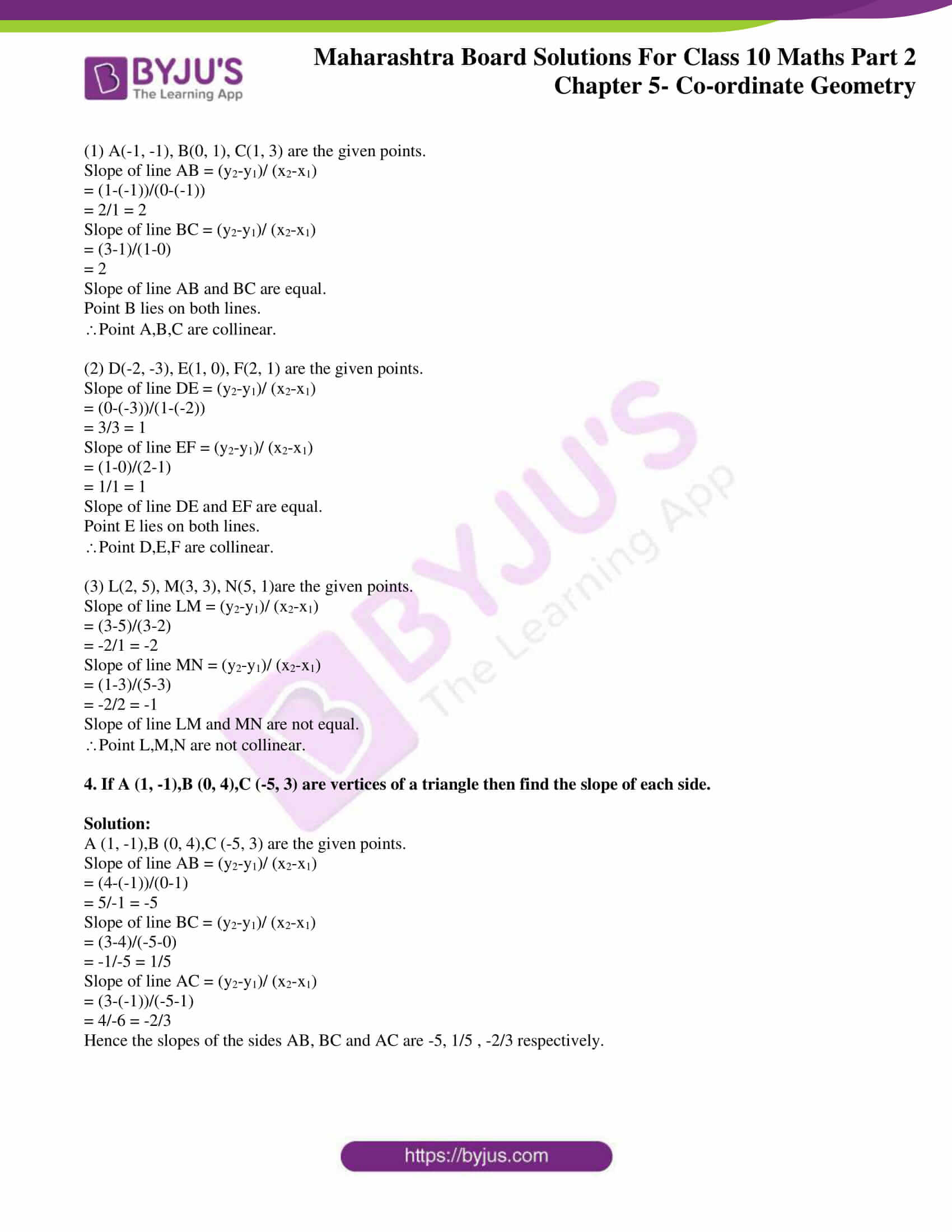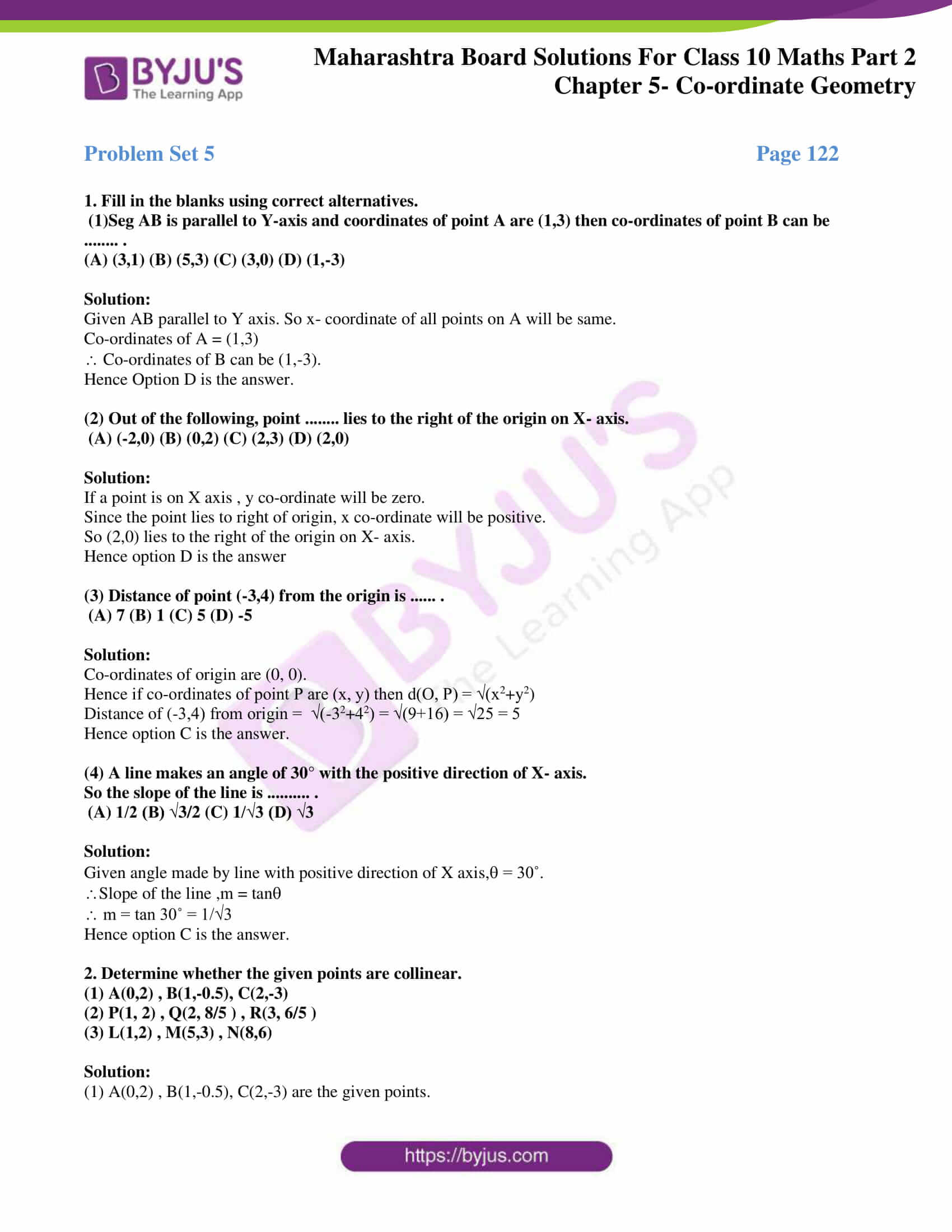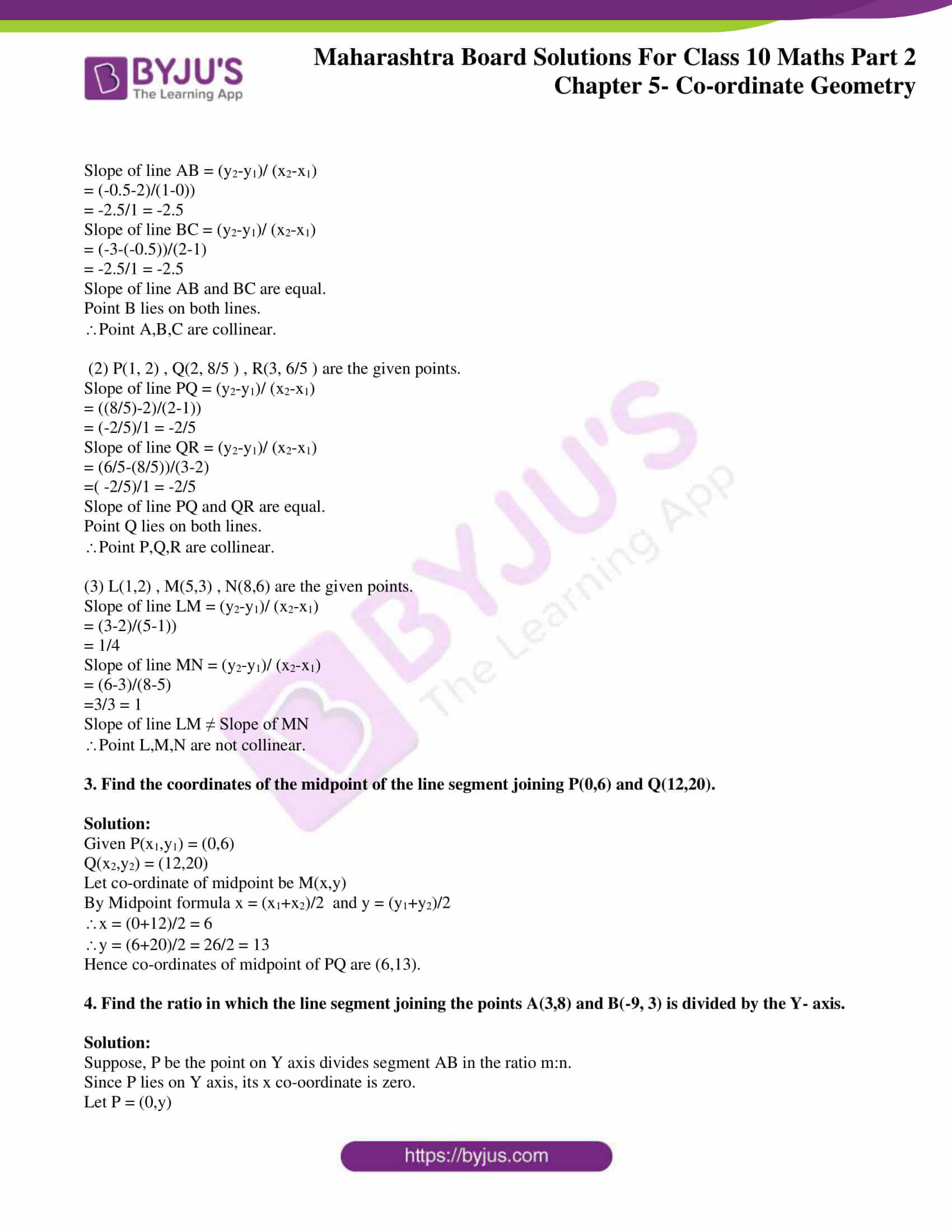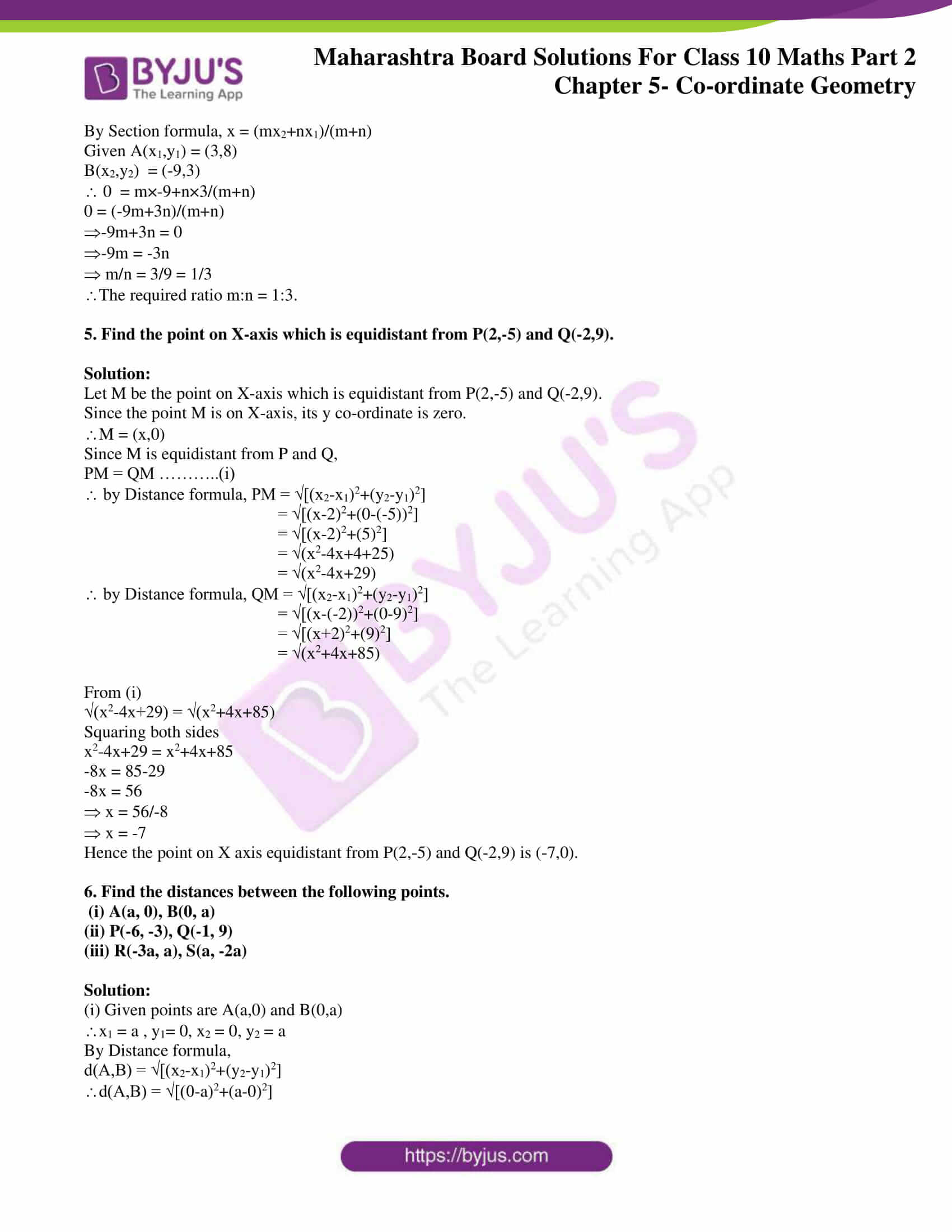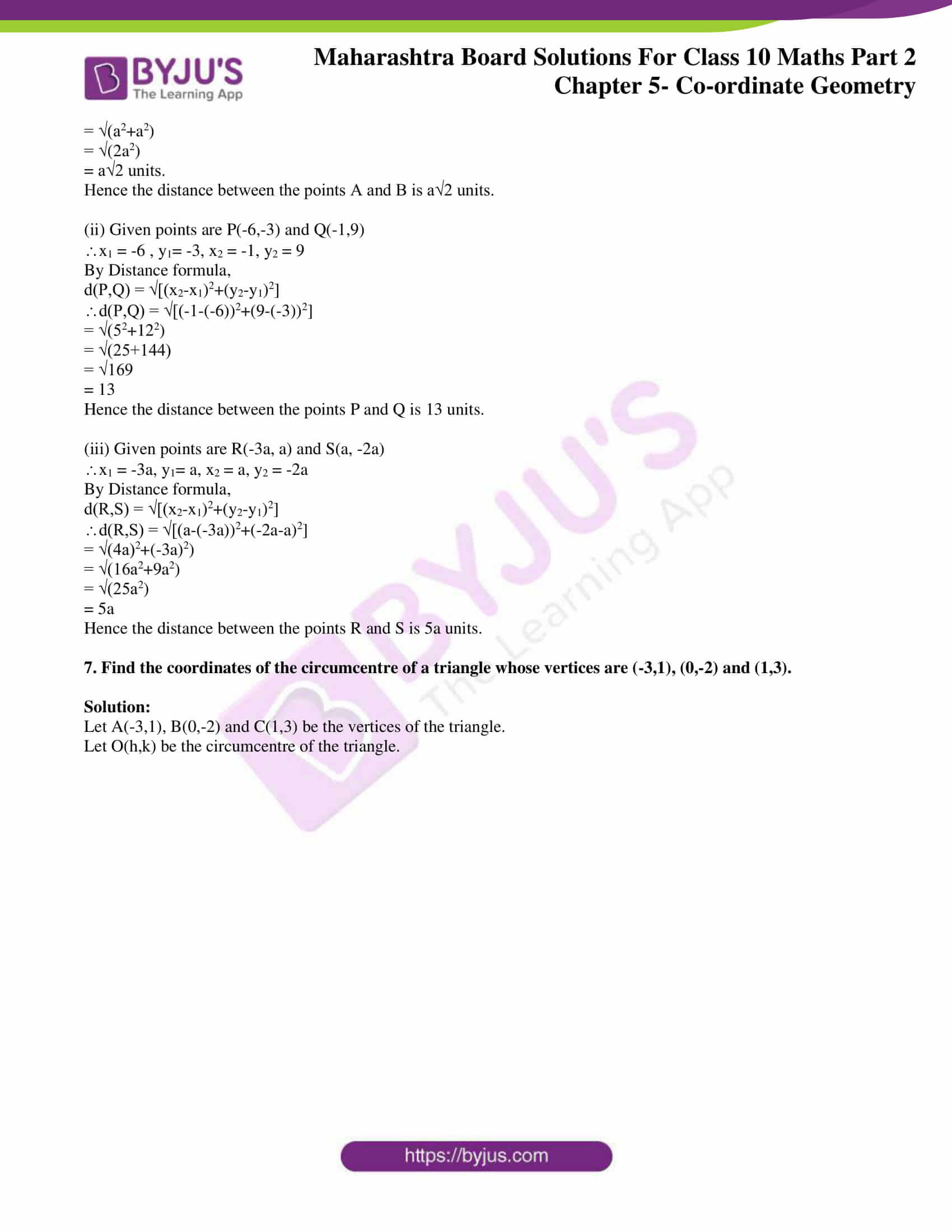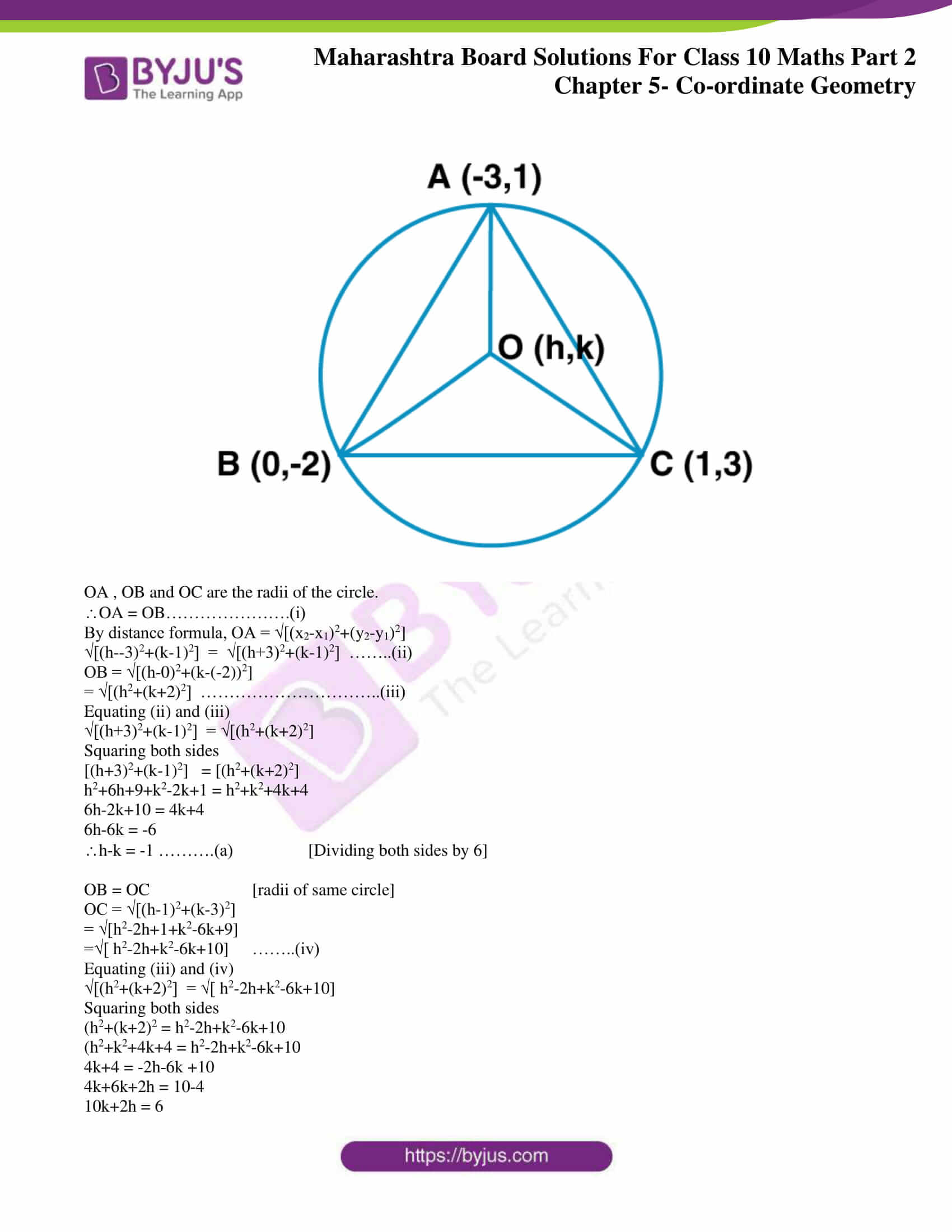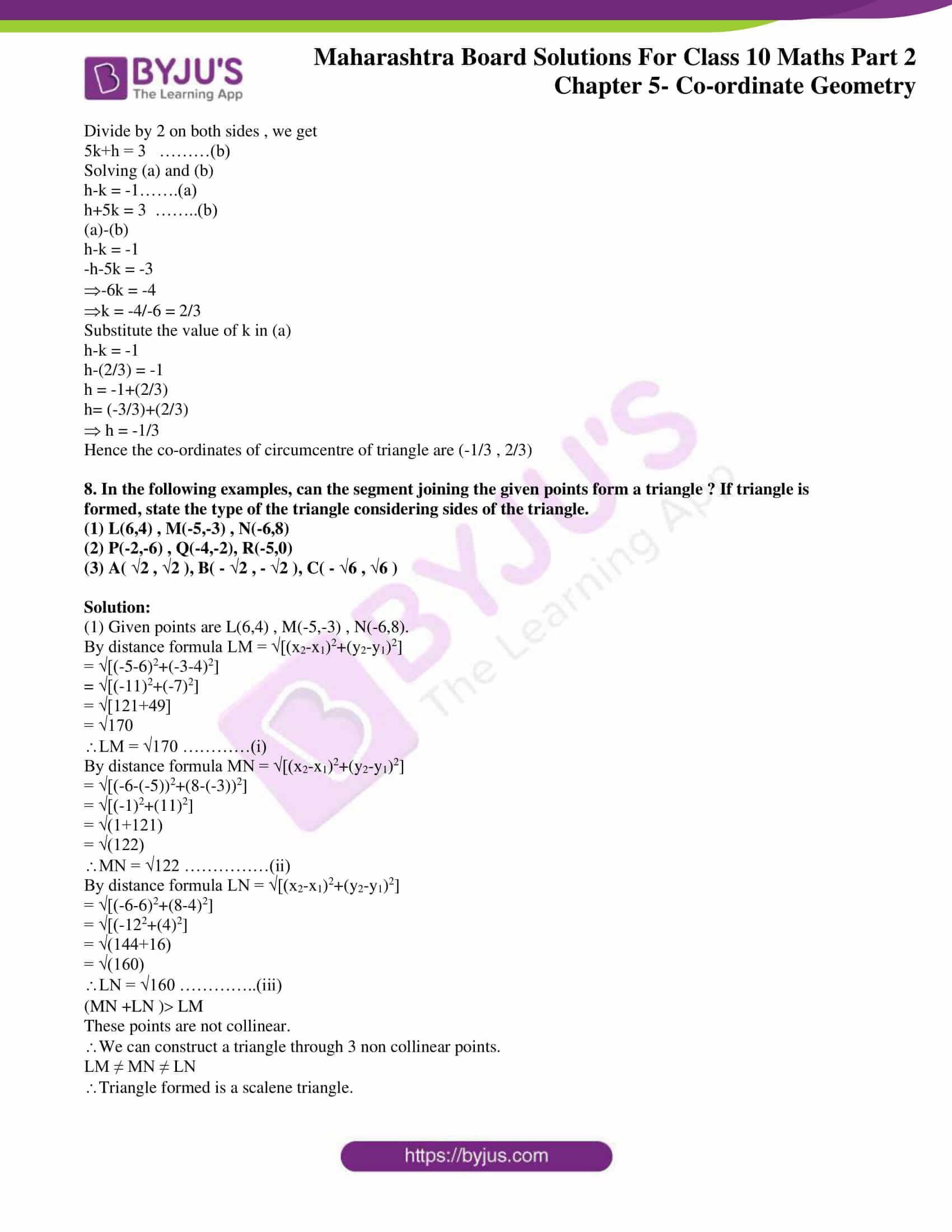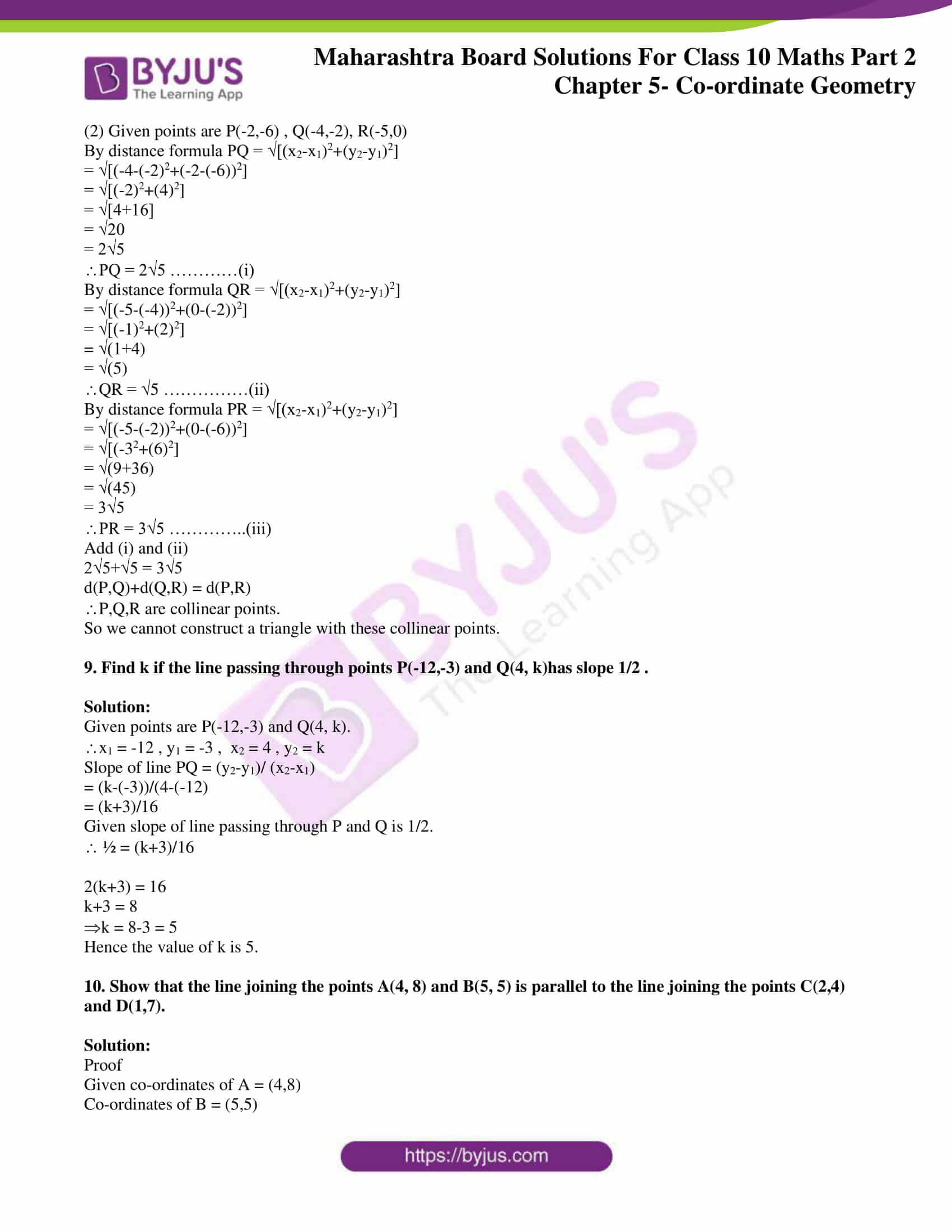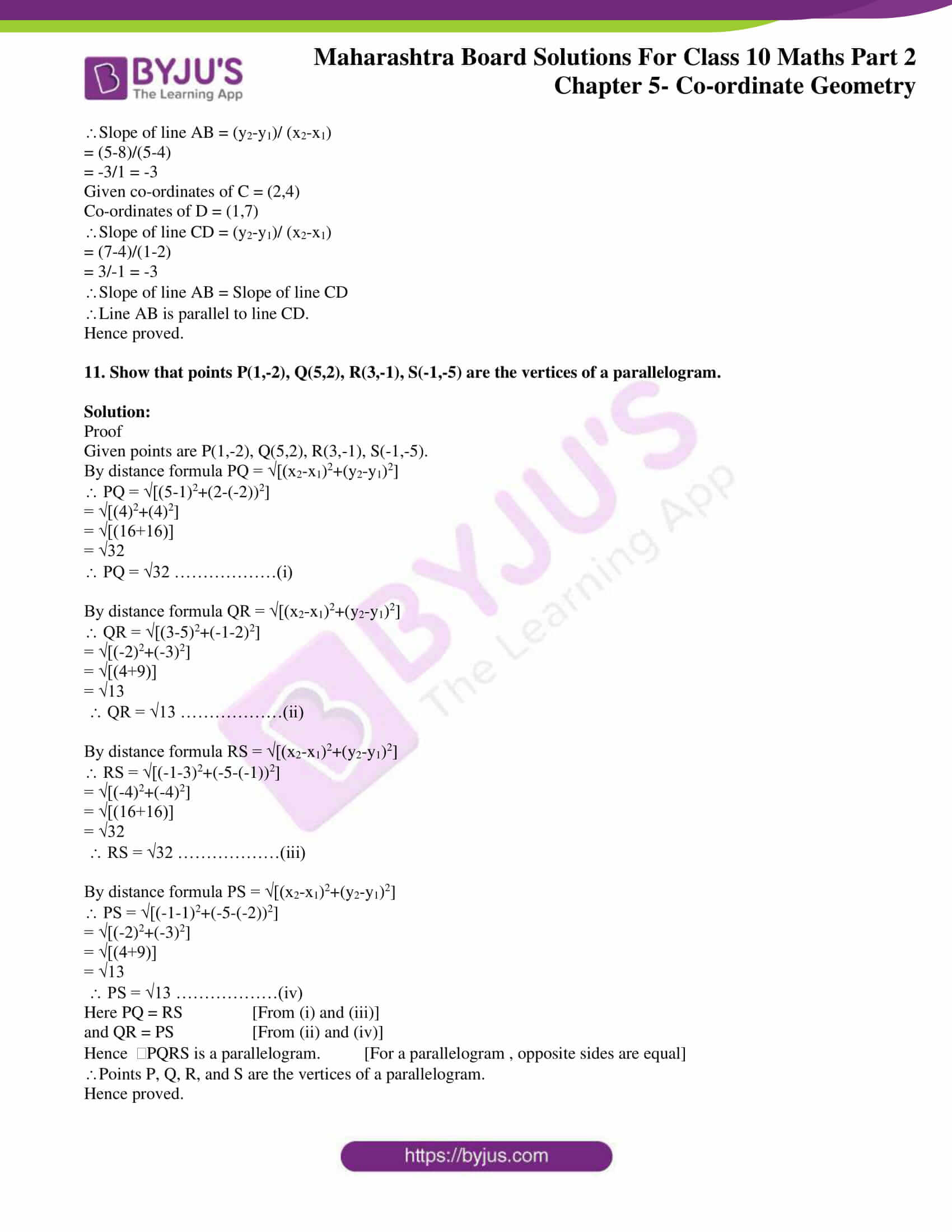## Access answers to Maths MSBSHSE Solutions For SSC Part 2 Chapter 5 – Coordinate Geometry

Practice Set 5.1 Page 107

1. Find the distance between each of the following pairs of points.

(1)A(2, 3), B(4, 1)

(2) P(-5, 7), Q(-1, 3)

(3) R(0, -3), S(0, 5/2 )

Solution:

(1) Let A(x1, y1) and B(x2 , y2) be the given points

By distance formula d(A,B) = √[(x2-x1)2+(y2-y1)2]

Here x1 = 2, y1 = 3 , x2 = 4, y2 = 1

d(A,B) = √[(4-2)2+(1-3)2]

= √[22+(-2)2]

= √8

= 2√2

Hence the distance between A and B is 2√2 units.

(2) Let P(x1, y1) and Q(x2 , y2) be the given points

By distance formula d(P,Q) = √[(x2-x1)2+(y2-y1)2]

Here x1 = -5, y1 = 7 , x2 = -1, y2 = 3

d(A,B) = √[(-1-(-5))2+(3-7)2]

= √[42+(-4)2]

= √32

= 4√2

Hence the distance between P and Q is 4√2 units.

(3) Let R(x1, y1) and S(x2 , y2) be the given points

By distance formula d(R,S) = √[(x2-x1)2+(y2-y1)2]

Here x1 = 0, y1 = -3 , x2 = 0, y2 = 5/2

d(A,B) = √[(0-0)2+((5/2) -(-3))2]

= √[02+(11/2)2]

= √(121/4)

= 11/2

Hence the distance between P and Q is 11/2 units.

2. Determine whether the points are collinear.

(1) A(1, -3), B(2, -5), C(-4, 7)

(2) L(-2, 3), M(1, -3), N(5, 4)

Solution:

(1) If the sum of any two distances out of d(A, B), d(B, C) and d(A, C) is equal to the third , then the three points A, B and C are collinear.

we will find d(A, B), d(B, C) and d(A, C).

Co-ordinates of A = (1,-3)

Co-ordinates of B = (2,-5)

Co-ordinates of C = (-4,7)

By distance formula d(A,B) = √[(x2-x1)2+(y2-y1)2]

d(A,B) = √[(2-1)2+(-5-(-3))2]

d(A,B) = √[(1)2+(-2)2]

d(A,B) = √(1+4)

d(A,B) = √5 ……………(i)

By distance formula d(B,C) = √[(x2-x1)2+(y2-y1)2]

d(B,C) = √[(-4-2)2+(7-(-5))2]

d(B,C) = √[(-6)2+(12)2]

d(B,C) = √(36+144)

d(B,C) = √180

d(B,C) = 6√5 ………….(ii)

By distance formula d(A,C) = √[(x2-x1)2+(y2-y1)2]

d(A,C) = √[(-4-1)2+(7-(-3))2]

d(A,C) = √[(-5)2+(10)2]

d(A,C) = √(25+100)

d(A,C) = √125

d(A,C) = 5√5………….(iii)

From (i) , (ii) and (iii)

√5 +5√5 = 6√5

d(A,C)+d(A,B) = d(B,C)

Hence Points A, B, C are collinear.

(2) If the sum of any two distances out of d(L, M), d(M, N) and d(L, N) is equal to the third , then the three points L, M and N are collinear.

we will find d(L, M), d(M, N) and d(L, N).

Co-ordinates of L = (-2, 3)

Co-ordinates of M = (1, -3)

Co-ordinates of N = (5, 4)

By distance formula d(L,M) = √[(x2-x1)2+(y2-y1)2]

d(L,M) = √[(1-(-2))2+(-3-3)2]

d(L,M) = √[32+(-6)2]

d(L,M) = √(9+36)

d(L,M) = √45

d(L,M) = 3√5……..(i)

By distance formula d(M,N) = √[(x2-x1)2+(y2-y1)2]

d(M,N) = √[(5-1)2+(4-(-3))2]

d(M,N) = √[42+72]

d(M,N) = √(16+49)

d(M,N)= √65……..(ii)

By distance formula d(L,N) = √[(x2-x1)2+(y2-y1)2]

d(L,N) = √[(5-(-2))2+(4-3)2]

d(L,N) = √[72+12]

d(L,N) = √(49+1)

d(L,M) = √50

d(L,M) =5 √2……..(iii)

d(L,M)+d(L,N) = 3√5+√50 ≠ √65

d(L,M)+d(L,N) ≠ d(M,N)

Points L,M,N are not collinear.

3. Find the point on the X-axis which is equidistant from A(-3, 4) and B(1, -4).

Solution:

Let C be the point on X axis equidistant from A(-3,4) and B(1,-4).

Since C lies on X axis, the Y co-ordinate of C is 0.

Let C = (x,0)

Co-ordinates of A = (-3, 4)

Co-ordinates of B = (1, -4)

Since C is equidistant from A and B ,

AC = BC

By distance formula, d(A,C) = √[(x2-x1)2+(y2-y1)2]

d(A,C) = √[(x-(-3))2+(0-4)2]

d(A,C) = √[(x+3)2+(4)2]

d(A,C) = √[(x+3)2+16] ……(i)

By distance formula, d(B,C) = √[(x2-x1)2+(y2-y1)2]

d(B,C) = √[(x-1)2+(0-(-4))2]

d(B,C) = √[(x-1)2+(4)2]

d(B,C) = √[(x-1)2+16] ……(ii)

Equating (i) and (ii) [∵AC = BC]

√[(x+3)2+16] = √[(x-1)2+16]

Squaring both sides

(x+3)2+16 = (x-1)2+16

x2+6x+9+16 = x2-2x+1+16

x2+6x+25 = x2-2x+17

8x = -8

x = -8/8 = -1

Hence the point on X axis which is equidistant from A and B is C(-1,0)

4. Verify that points P(-2, 2), Q(2, 2) and R(2, 7) are vertices of a right angled triangle.

Solution:

Given P(-2, 2), Q(2, 2) and R(2, 7).

If the square of the length of the longest side of a triangle is equal to the sum of the squares of the other two sides, then the triangle is a right triangle.

By distance formula , distance between two points = √[(x2-x1)2+(y2-y1)2]

PQ = √[(2-(-2))2+(2-2)2]

PQ = √[(42+02]

PQ = √16

PQ = 4 …..(i)

QR = √[(2-2)2+(7-2)2]

QR = √[(0)2+(5)2]

QR = √25

QR = 5 …….(ii)

PR = √[(2-(-2))2+(7-2)2]

PR = √[42+52]

PR = √16+25

PR = √41…….(iii)

PQ2+QR2 = 42+52

= 16+25

=41

PR2 = 41

PQ2+QR2 = PR2

PQR is a right triangle.

P,Q,R are the vertices of a right angled triangle.

Practice Set 5.2 Page 115

1. Find the coordinates of point P if P divides the line segment joining the points A(-1,7) and B(4,-3) in the ratio 2:3.

Solution:

Let the co-ordinates of P be (x, y).

A(-1,7) and B(4,-3) are the given points.

x1 = -1 , y1 = 7 , x2 = 4 , y2 = -3 , m = 2 and n = 3

By Section formula x = (mx2+nx1)/(m+n)

x = (2×4+3×-1)/(2+3)

x = (8-3)/5

x = 5/5

x= 1

By Section formula y = (my2+ny1)/(m+n)

y = (2×-3+3×7)/5

y = (-6+21)/5

y = 15/5

y = 3

Hence the co-ordinate of point P is (1,3).

2. In each of the following examples find the co-ordinates of point A which divides segment PQ in the ratio a:b.

(1) P(-3, 7), Q(1, -4), a:b = 2:1

(2) P(-2, -5), Q(4, 3), a:b = 3:4

(3) P(2, 6), Q(-4, 1), a:b = 1:2

Solution:

(1) Let the co-ordinates of A be (x, y).

P(-3, 7) and Q(1, -4) are the given points.

x1 = -3 , y1 = 7 , x2 = 1 , y2 = -4 , m = 2 and n = 1

By Section formula x = (mx2+nx1)/(m+n)

x = (2×1+1×-3)/(2+1)

x = -1/3

By Section formula y = (my2+ny1)/(m+n)

y = (2×-4+1×7)/3

y = (-8+7)/3

y = -1/3

Hence the co-ordinates of A is (-1/3 , -1/3)

(2) Let the co-ordinates of A be (x, y).

P(-2, -5), and Q(4, 3), are the given points.

x1 = -2 , y1 = -5 , x2 = 4 , y2 = 3 , m = 3 and n = 4

By Section formula x = (mx2+nx1)/(m+n)

x = (3×4+4×-2)/(3+4)

x = (12+-8)/(3+4)

x = 4/7

By Section formula y = (my2+ny1)/(m+n)

y = (3×3+4×-5)/(3+4)

y = (9-20)/7

y = -11/7

Hence the co-ordinates of A is (4/7 , -11/7)

(3) Let the co-ordinates of A be (x, y).

P(2, 6), and Q(-4, 1), are the given points.

x1 = 2 , y1 = 6 , x2 = -4, y2 = 1 , m =1 and n = 2

By section formula x = (mx2+nx1)/(m+n)

x = (1×-4+2×2)/(1+2)

x = (-4+4)/(1+2)

x = 0

By Section formula y = (my2+ny1)/(m+n)

y = (1×1+2×6)/(1+2)

y = (1+12)/3

y = 13/3

Hence the co-ordinates of A is (0 , 13/3)

3. Find the ratio in which point T(-1, 6)divides the line segment joining the points P(-3, 10) and Q(6, -8).

Solution:

Let, point T(-1,6) divides segment PQ in the ratio m:n

Given P(-3, 10) and Q(6, -8).

x = -1 , y = 6

x1 = -3 , y1 = 10

x2 = 6 , y2 = -8

By Section formula, x = (mx2+nx1)/(m+n)

-1 = m×6+n×-3/(m+n)

-1 = 6m-3n/(m+n)

-m+-n = 6m-3n

2n = 7m

m/n = 2/7

m:n = 2:7

Point T divides PQ is the ratio 2:7.

4. Point P is the centre of the circle and AB is a diameter . Find the coordinates of point B if coordinates of point A and P are (2, -3) and (-2, 0) respectively.

Solution: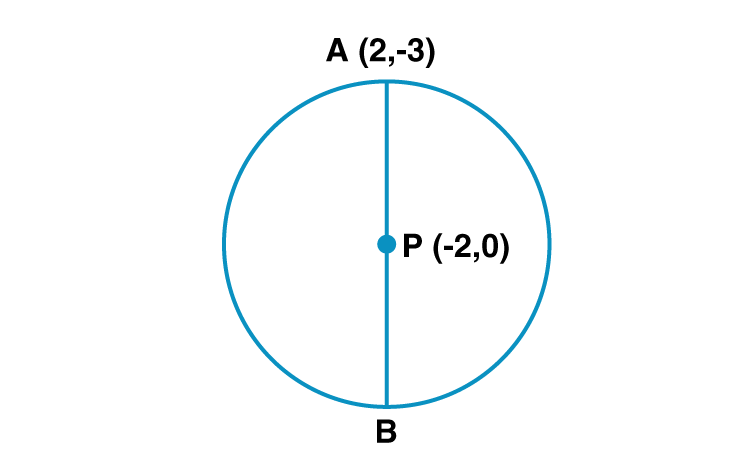Given co-ordinates of P = (-2,0) = (x,y)

Co-ordinates of A = (2,-3) = (x1, y1)

Let co-ordinates of B = (x2,y2)

Since P is the midpoint of diameter AB ,

By midpoint formula x = (x1+x2)/2

-2 = 2+x2/2

x2 = -4-2

x2 = -6

y = (y1+y2)/2

0 = (-3+y2)/2

-3+y2 = 0

y2 = 3

Hence the co-ordinates of point B is (-6,3).

5. Find the ratio in which point P(k, 7) divides the segment joining A(8, 9) and B(1, 2). Also find k .

Solution:

Let P (x, y) , A (x1, y1) and B (x2, y2) and be the given points.

Here, x = k, y = 7, x1 = 8, y1 = 9, x2 = 1, y2 = 2,

By Section formula y = (my2+ny1)/(m+n)

7 = m×2+n×9/(m+n)

7 = 2m+9n/(m+n)

7m+7n = 2m+9n

7m-2n = 9n-7n

5m = 2n

m/n = 2/5

Hence m:n = 2:5.

By Section formula, x = (mx2+nx1)/(m+n)

k = (2×1+5×8)/2+5

k = (2+40)/7

k = 42/7 = 6

Hence value of k is 6.

Hence P(6,7) divides the segment in the ration 2:5.

6. Find the coordinates of midpoint of the segment joining the points (22, 20) and (0, 16).

Solution:

Let (x1,y1) = (22,20)

(x2,y2) = (0,16)

Let co-ordinate of midpoint be A(x,y)

By Midpoint formula x = (x1+x2)/2 and y = (y1+y2)/2

x = (22+0)/2 = 11

y = (20+16)/2 = 36/2 = 18

Hence co-ordinates of midpoint are (11,18).

Practice Set 5.3 Page 121

1. Angles made by the line with the positive direction of X-axis are given. Find the slope of these lines.

(1) 45°

(2) 60°

(3) 90°

Solution:

(1) Given angle made by line with positive direction of X axis, = 45˚.

Slope of the line ,m = tan

m = tan 45˚ = 1

Hence slope of the line is 1.

(2) Given angle made by line with positive direction of X axis, = 60˚.

Slope of the line ,m = tan

m = tan 60˚ = √3

Hence slope of the line is √3.

(3) Given angle made by line with positive direction of X axis, = 90˚.

Slope of the line ,m = tan

m = tan 90˚ = not defined

Hence slope of the line cannot be determined.

2. Find the slopes of the lines passing through the given points.

(1) A (2, 3) , B (4, 7)

(2) P (-3, 1) , Q (5, -2)

(3) C (5, -2) , D (7, 3)

(4) L (-2, -3) , M (-6, -8)

(5) E(-4, -2) , F (6, 3)

(6) T (0, -3) , S (0, 4)

Solution:

(1) Given A(2,3) and B(4,7)

x1 = 2

y1 = 3

x2 = 4

y2 = 7

Slope of the line AB = (y2-y1)/ (x2-x1)

= (7-3)/(4-2)

= 4/2 = 2

Hence Slope of line AB is 2.

(2) Given P(-3,1) and Q(5,-2)

x1 = -3

y1 = 1

x2 = 5

y2 = -2

Slope of the line PQ = (y2-y1)/ (x2-x1)

= (-2-1)/(5-(-3))

= -3/8

Hence Slope of line PQ is -3/8.

(3) Given C(5,-2) and D(7,3)

x1 = 5

y1 = -2

x2 = 7

y2 = 3

Slope of the line CD = (y2-y1)/ (x2-x1)

= (3-(-2))/(7-5)

= 5/2

Hence Slope of line CD is 5/2.

(4) Given L(-2,-3) and M(-6,-8)

x1 = -2

y1 = -3

x2 = -6

y2 = -8

Slope of the line LM = (y2-y1)/ (x2-x1)

= (-8-(-3))/(-6-(-2))

= -5/-4 = 5/4

Hence Slope of line LM is 5/4.

(5) Given E(-4,-2) and F(6,3)

x1 = -4

y1 = -2

x2 = 6

y2 = 3

Slope of the line EF = (y2-y1)/ (x2-x1)

= (3-(-2))/(6-(-4))

= 5/10 = 1/5

Hence Slope of line EF is 1/2.

(6) Given T(0,-3) and S(0,4)

x1 = 0

y1 = -3

x2 = 0

y2 = 4

Slope of the line TS = (y2-y1)/ (x2-x1)

= (4-(-3))/(0-0)

= 7/0 = not defined

Hence Slope of line TS cannot be determined.

3. Determine whether the following points are collinear.

(1) A(-1, -1), B(0, 1), C(1, 3)

(2) D(-2, -3), E(1, 0), F(2, 1)

(3) L(2, 5), M(3, 3), N(5, 1)

Solution:

(1) A(-1, -1), B(0, 1), C(1, 3) are the given points.

Slope of line AB = (y2-y1)/ (x2-x1)

= (1-(-1))/(0-(-1))

= 2/1 = 2

Slope of line BC = (y2-y1)/ (x2-x1)

= (3-1)/(1-0)

= 2

Slope of line AB and BC are equal.

Point B lies on both lines.

Point A,B,C are collinear.

(2) D(-2, -3), E(1, 0), F(2, 1) are the given points.

Slope of line DE = (y2-y1)/ (x2-x1)

= (0-(-3))/(1-(-2))

= 3/3 = 1

Slope of line EF = (y2-y1)/ (x2-x1)

= (1-0)/(2-1)

= 1/1 = 1

Slope of line DE and EF are equal.

Point E lies on both lines.

Point D,E,F are collinear.

(3) L(2, 5), M(3, 3), N(5, 1)are the given points.

Slope of line LM = (y2-y1)/ (x2-x1)

= (3-5)/(3-2)

= -2/1 = -2

Slope of line MN = (y2-y1)/ (x2-x1)

= (1-3)/(5-3)

= -2/2 = -1

Slope of line LM and MN are not equal.

Point L,M,N are not collinear.

4. If A (1, -1),B (0, 4),C (-5, 3) are vertices of a triangle then find the slope of each side.

Solution:

A (1, -1),B (0, 4),C (-5, 3) are the given points.

Slope of line AB = (y2-y1)/ (x2-x1)

= (4-(-1))/(0-1)

= 5/-1 = -5

Slope of line BC = (y2-y1)/ (x2-x1)

= (3-4)/(-5-0)

= -1/-5 = 1/5

Slope of line AC = (y2-y1)/ (x2-x1)

= (3-(-1))/(-5-1)

= 4/-6 = -2/3

Hence the slopes of the sides AB, BC and AC are -5, 1/5 , -2/3 respectively.

Problem Set 5 Page 122

1. Fill in the blanks using correct alternatives.

(1)Seg AB is parallel to Y-axis and coordinates of point A are (1,3) then co-ordinates of point B can be …….. .

(A) (3,1) (B) (5,3) (C) (3,0) (D) (1,-3)

Solution:

Given AB parallel to Y axis. So x- coordinate of all points on A will be same.

Co-ordinates of A = (1,3)

Co-ordinates of B can be (1,-3).

Hence Option D is the answer.

(2) Out of the following, point …….. lies to the right of the origin on X- axis.

(A) (-2,0) (B) (0,2) (C) (2,3) (D) (2,0)

Solution:

If a point is on X axis , y co-ordinate will be zero.

Since the point lies to right of origin, x co-ordinate will be positive.

So (2,0) lies to the right of the origin on X- axis.

Hence option D is the answer

(3) Distance of point (-3,4) from the origin is …… .

(A) 7 (B) 1 (C) 5 (D) -5

Solution:

Co-ordinates of origin are (0, 0).

Hence if co-ordinates of point P are (x, y) then d(O, P) = √(x2+y2)

Distance of (-3,4) from origin = √(-32+42) = √(9+16) = √25 = 5

Hence option C is the answer.

(4) A line makes an angle of 30° with the positive direction of X- axis.

So the slope of the line is ………. .

1. 1/2 (B) √3/2 (C) 1/√3 (D) √3

Solution:

Given angle made by line with positive direction of X axis, = 30˚.

Slope of the line ,m = tan

m = tan 30˚ = 1/√3

Hence option C is the answer.

2. Determine whether the given points are collinear.

(1) A(0,2) , B(1,-0.5), C(2,-3)

(2) P(1, 2) , Q(2, 8/5 ) , R(3, 6/5 )

(3) L(1,2) , M(5,3) , N(8,6)

Solution:

(1) A(0,2) , B(1,-0.5), C(2,-3) are the given points.

Slope of line AB = (y2-y1)/ (x2-x1)

= (-0.5-2)/(1-0))

= -2.5/1 = -2.5

Slope of line BC = (y2-y1)/ (x2-x1)

= (-3-(-0.5))/(2-1)

= -2.5/1 = -2.5

Slope of line AB and BC are equal.

Point B lies on both lines.

Point A,B,C are collinear.

(2) P(1, 2) , Q(2, 8/5 ) , R(3, 6/5 ) are the given points.

Slope of line PQ = (y2-y1)/ (x2-x1)

= ((8/5)-2)/(2-1))

= (-2/5)/1 = -2/5

Slope of line QR = (y2-y1)/ (x2-x1)

= (6/5-(8/5))/(3-2)

=( -2/5)/1 = -2/5

Slope of line PQ and QR are equal.

Point Q lies on both lines.

Point P,Q,R are collinear.

(3) L(1,2) , M(5,3) , N(8,6) are the given points.

Slope of line LM = (y2-y1)/ (x2-x1)

= (3-2)/(5-1))

= 1/4

Slope of line MN = (y2-y1)/ (x2-x1)

= (6-3)/(8-5)

=3/3 = 1

Slope of line LM ≠ Slope of MN

Point L,M,N are not collinear.

3. Find the coordinates of the midpoint of the line segment joining P(0,6) and Q(12,20).

Solution:

Given P(x1,y1) = (0,6)

Q(x2,y2) = (12,20)

Let co-ordinate of midpoint be M(x,y)

By Midpoint formula x = (x1+x2)/2 and y = (y1+y2)/2

x = (0+12)/2 = 6

y = (6+20)/2 = 26/2 = 13

Hence co-ordinates of midpoint of PQ are (6,13).

4. Find the ratio in which the line segment joining the points A(3,8) and B(-9, 3) is divided by the Y- axis.

Solution:

Suppose, P be the point on Y axis divides segment AB in the ratio m:n.

Since P lies on Y axis, its x co-oordinate is zero.

Let P = (0,y)

By Section formula, x = (mx2+nx1)/(m+n)

Given A(x1,y1) = (3,8)

B(x2,y2) = (-9,3)

0 = m×-9+n×3/(m+n)

0 = (-9m+3n)/(m+n)

-9m+3n = 0

-9m = -3n

m/n = 3/9 = 1/3

The required ratio m:n = 1:3.

5. Find the point on X-axis which is equidistant from P(2,-5) and Q(-2,9).

Solution:

Let M be the point on X-axis which is equidistant from P(2,-5) and Q(-2,9).

Since the point M is on X-axis, its y co-ordinate is zero.

M = (x,0)

Since M is equidistant from P and Q,

PM = QM ………..(i)

by Distance formula, PM = √[(x2-x1)2+(y2-y1)2]

= √[(x-2)2+(0-(-5))2]

= √[(x-2)2+(5)2]

= √(x2-4x+4+25)

= √(x2-4x+29)

by Distance formula, QM = √[(x2-x1)2+(y2-y1)2]

= √[(x-(-2))2+(0-9)2]

= √[(x+2)2+(9)2]

= √(x2+4x+85)

From (i)

√(x2-4x+29) = √(x2+4x+85)

Squaring both sides

x2-4x+29 = x2+4x+85

-8x = 85-29

-8x = 56

x = 56/-8

x = -7

Hence the point on X axis equidistant from P(2,-5) and Q(-2,9) is (-7,0).

6. Find the distances between the following points.

(i) A(a, 0), B(0, a)

(ii) P(-6, -3), Q(-1, 9)

(iii) R(-3a, a), S(a, -2a)

Solution:

(i) Given points are A(a,0) and B(0,a)

x1 = a , y1= 0, x2 = 0, y2 = a

By Distance formula,

d(A,B) = √[(x2-x1)2+(y2-y1)2]

d(A,B) = √[(0-a)2+(a-0)2]

= √(a2+a2)

= √(2a2)

= a√2 units.

Hence the distance between the points A and B is a√2 units.

(ii) Given points are P(-6,-3) and Q(-1,9)

x1 = -6 , y1= -3, x2 = -1, y2 = 9

By Distance formula,

d(P,Q) = √[(x2-x1)2+(y2-y1)2]

d(P,Q) = √[(-1-(-6))2+(9-(-3))2]

= √(52+122)

= √(25+144)

= √169

= 13

Hence the distance between the points P and Q is 13 units.

(iii) Given points are R(-3a, a) and S(a, -2a)

x1 = -3a, y1= a, x2 = a, y2 = -2a

By Distance formula,

d(R,S) = √[(x2-x1)2+(y2-y1)2]

d(R,S) = √[(a-(-3a))2+(-2a-a)2]

= √(4a)2+(-3a)2)

= √(16a2+9a2)

= √(25a2)

= 5a

Hence the distance between the points R and S is 5a units.

7. Find the coordinates of the circumcentre of a triangle whose vertices are (-3,1), (0,-2) and (1,3).

Solution:

Let A(-3,1), B(0,-2) and C(1,3) be the vertices of the triangle.

Let O(h,k) be the circumcentre of the triangle.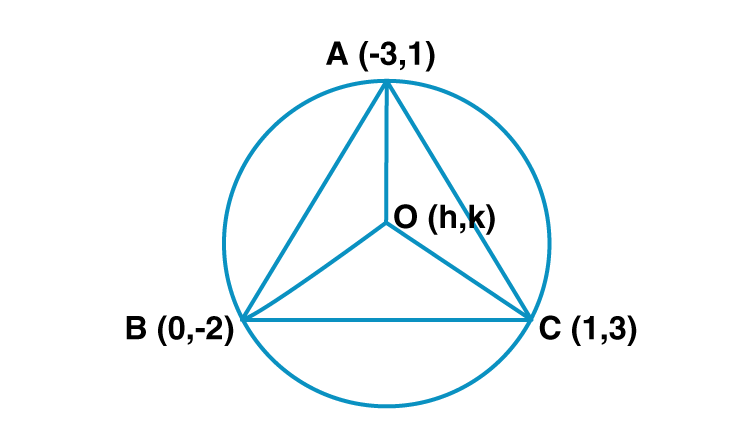OA , OB and OC are the radii of the circle.

OA = OB………………….(i)

By distance formula, OA = √[(x2-x1)2+(y2-y1)2]

√[(h–3)2+(k-1)2] = √[(h+3)2+(k-1)2] ……..(ii)

OB = √[(h-0)2+(k-(-2))2]

= √[(h2+(k+2)2] …………………………..(iii)

Equating (ii) and (iii)

√[(h+3)2+(k-1)2] = √[(h2+(k+2)2]

Squaring both sides

[(h+3)2+(k-1)2] = [(h2+(k+2)2]

h2+6h+9+k2-2k+1 = h2+k2+4k+4

6h-2k+10 = 4k+4

6h-6k = -6

h-k = -1 ……….(a) [Dividing both sides by 6]

OB = OC [radii of same circle]

OC = √[(h-1)2+(k-3)2]

= √[h2-2h+1+k2-6k+9]

=√[ h2-2h+k2-6k+10] ……..(iv)

Equating (iii) and (iv)

√[(h2+(k+2)2] = √[ h2-2h+k2-6k+10]

Squaring both sides

(h2+(k+2)2 = h2-2h+k2-6k+10

(h2+k2+4k+4 = h2-2h+k2-6k+10

4k+4 = -2h-6k +10

4k+6k+2h = 10-4

10k+2h = 6

Divide by 2 on both sides , we get

5k+h = 3 ………(b)

Solving (a) and (b)

h-k = -1…….(a)

h+5k = 3 ……..(b)

(a)-(b)

h-k = -1

-h-5k = -3

-6k = -4

k = -4/-6 = 2/3

Substitute the value of k in (a)

h-k = -1

h-(2/3) = -1

h = -1+(2/3)

h= (-3/3)+(2/3)

h = -1/3

Hence the co-ordinates of circumcentre of triangle are (-1/3 , 2/3)

8. In the following examples, can the segment joining the given points form a triangle ? If triangle is formed, state the type of the triangle considering sides of the triangle.

(1) L(6,4) , M(-5,-3) , N(-6,8)

(2) P(-2,-6) , Q(-4,-2), R(-5,0)

(3) A( √2 , √2 ), B( – √2 , – √2 ), C( – √6 , √6 )

Solution:

(1) Given points are L(6,4) , M(-5,-3) , N(-6,8).

By distance formula LM = √[(x2-x1)2+(y2-y1)2]

= √[(-5-6)2+(-3-4)2]

= √[(-11)2+(-7)2]

= √[121+49]

= √170

LM = √170 …………(i)

By distance formula MN = √[(x2-x1)2+(y2-y1)2]

= √[(-6-(-5))2+(8-(-3))2]

= √[(-1)2+(11)2]

= √(1+121)

= √(122)

MN = √122 ……………(ii)

By distance formula LN = √[(x2-x1)2+(y2-y1)2]

= √[(-6-6)2+(8-4)2]

= √[(-122+(4)2]

= √(144+16)

= √(160)

LN = √160 …………..(iii)

(MN +LN ) LM

These points are not collinear.

We can construct a triangle through 3 non collinear points.

LM ≠ MN ≠ LN

Triangle formed is a scalene triangle.

(2) Given points are P(-2,-6) , Q(-4,-2), R(-5,0)

By distance formula PQ = √[(x2-x1)2+(y2-y1)2]

= √[(-4-(-2)2+(-2-(-6))2]

= √[(-2)2+(4)2]

= √[4+16]

= √20

= 2√5

PQ = 2√5 …………(i)

By distance formula QR = √[(x2-x1)2+(y2-y1)2]

= √[(-5-(-4))2+(0-(-2))2]

= √[(-1)2+(2)2]

= √(1+4)

= √(5)

QR = √5 ……………(ii)

By distance formula PR = √[(x2-x1)2+(y2-y1)2]

= √[(-5-(-2))2+(0-(-6))2]

= √[(-32+(6)2]

= √(9+36)

= √(45)

= 3√5

PR = 3√5 …………..(iii)

2√5+√5 = 3√5

d(P,Q)+d(Q,R) = d(P,R)

P,Q,R are collinear points.

So we cannot construct a triangle with these collinear points.

9. Find k if the line passing through points P(-12,-3) and Q(4, k)has slope 1/2 .

Solution:

Given points are P(-12,-3) and Q(4, k).

x1 = -12 , y1 = -3 , x2 = 4 , y2 = k

Slope of line PQ = (y2-y1)/ (x2-x1)

= (k-(-3))/(4-(-12)

= (k+3)/16

Given slope of line passing through P and Q is 1/2.

½ = (k+3)/16

2(k+3) = 16

k+3 = 8

k = 8-3 = 5

Hence the value of k is 5.

10. Show that the line joining the points A(4, 8) and B(5, 5) is parallel to the line joining the points C(2,4) and D(1,7).

Solution:

Proof

Given co-ordinates of A = (4,8)

Co-ordinates of B = (5,5)

Slope of line AB = (y2-y1)/ (x2-x1)

= (5-8)/(5-4)

= -3/1 = -3

Given co-ordinates of C = (2,4)

Co-ordinates of D = (1,7)

Slope of line CD = (y2-y1)/ (x2-x1)

= (7-4)/(1-2)

= 3/-1 = -3

Slope of line AB = Slope of line CD

Line AB is parallel to line CD.

Hence proved.

11. Show that points P(1,-2), Q(5,2), R(3,-1), S(-1,-5) are the vertices of a parallelogram.

Solution:

Proof

Given points are P(1,-2), Q(5,2), R(3,-1), S(-1,-5).

By distance formula PQ = √[(x2-x1)2+(y2-y1)2]

PQ = √[(5-1)2+(2-(-2))2]

= √[(4)2+(4)2]

= √[(16+16)]

= √32

PQ = √32 ………………(i)

By distance formula QR = √[(x2-x1)2+(y2-y1)2]

QR = √[(3-5)2+(-1-2)2]

= √[(-2)2+(-3)2]

= √[(4+9)]

= √13

QR = √13 ………………(ii)

By distance formula RS = √[(x2-x1)2+(y2-y1)2]

RS = √[(-1-3)2+(-5-(-1))2]

= √[(-4)2+(-4)2]

= √[(16+16)]

= √32

RS = √32 ………………(iii)

By distance formula PS = √[(x2-x1)2+(y2-y1)2]

PS = √[(-1-1)2+(-5-(-2))2]

= √[(-2)2+(-3)2]

= √[(4+9)]

= √13

PS = √13 ………………(iv)

Here PQ = RS [From (i) and (iii)]

and QR = PS [From (ii) and (iv)]

Hence PQRS is a parallelogram. [For a parallelogram , opposite sides are equal]

Points P, Q, R, and S are the vertices of a parallelogram.

Hence proved.

Coordinate Geometry is a branch of Mathematics, which deals with the position of points on a plane described by an ordered pair of numbers. This chapter deals with distance formula, centroid formula, section formula and midpoint formula. In this chapter, students learn how to find the slope of a line. Log on to BYJU’S to get more information on mathematical concepts and master Coordinate Geometry problems.

## Frequently Asked Questions on Maharashtra State Board Solutions for Class 10 Maths Part 2 Chapter 5 Coordinate Geometry

### Can we use these Maharashtra State Board Class 10 Maths Part 2 Chapter 5 Solutions to prepare for the exams?

Yes, these solutions will help to prepare for the board exams. Students are highly recommended to practise these solutions after revising the subjects, as they set the basis for the questions that could get asked in the board exams.

### How can students make the best use of these solutions?

Students are encouraged to practise these questions at first. Then they can refer back to the solutions to analyse their performance. This will also help the students to rectify the mistakes and avoid making them during the board exams.

### Is is possible to download these Maharashtra State Board Class 10 Maths Part 2 Chapter 5 Solutions?

Yes, the Maharashtra State Board Class 10 Maths Part 2 Chapter 5 Solutions can be downloaded. We have provided the solutions as a scrollable PDF and have also mentioned the clickable link for the students to access. Meanwhile, the questions and the solutions  are also available online on our webpage.